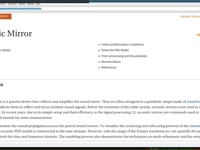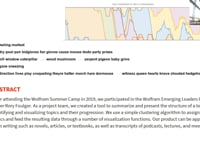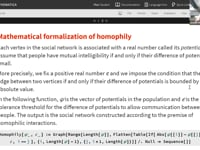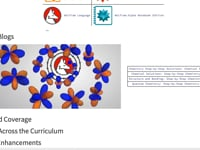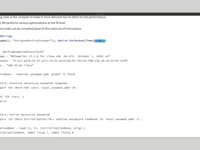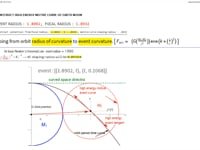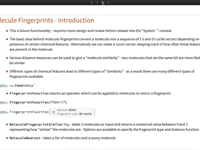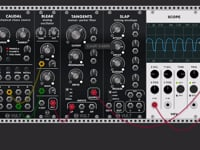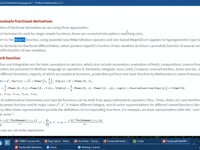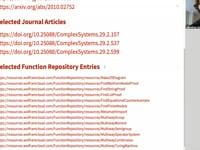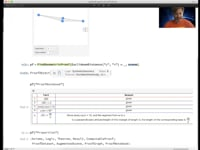Learn how »

# Fractional-Order Differentiation

This talk by Oleg Marichev and Paco Jain is devoted to the new operation FractionalD[f[z], {z,α}], which is presented in the Wolfram Function Repository. FractionalD is analytical by order α operator, which for integer-positive α coincides with the usual αth-order derivative, for integer-negative α coincides with repeatable α times indefinite integral and for α=0 coincides with the initial function. The building of such an operator was not an easy problem, because differentiation of basic Taylor series power function z^γ always gives a power function with constant coefficient and different power, but integration of z^γ can produce log(z) (for negative integer γ). We have solved this problem by an extension well known from literature generic result Γ(γ+1) z^(γα)/Γ(γα+1) for integro-differentiation of z^γ to value (1)(γ1) (ψ(γ)ψ(γα+1)+log(z))/((γ1)! Γ(γα+1)) z(γα), which should be applied for negative integer γ. More than 100 basic functions in Mathematica have representations as infinite sums ckz^(γ+k) with constant ck. It allowed us to build fractional derivatives of order α for more than 60,000 test cases, including basic functions and their compositions. Their values coincide with results based on the most popular RiemannLiouville approach to fractional integro-differentiation.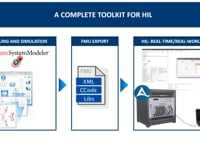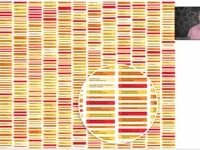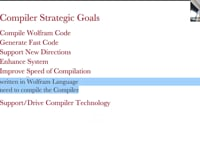The Wolfram Compiler61:14